# Goals

• Determine the properties of maximum error on segmenting
• Explore segmentation on complete data sets
• Look at segmentation as it relates to stop/track identification

# Creating segments

Location data is often generated as a sequence of points in time reflecting a geographical position at a moment in time. These coordinates reflect someone’s continuous location history with a set of discrete points which make calculations on underlying trajectories costly and conceptually difficult. If the data can be reduced in size without sacrificing information, it is possible to simultaneously reduce both the computational complexity as well as the number of assumptions that must be made about individual locations. One method of data reduction is by partitioning trajectories into straight line segments that sufficiently represent the path. These line segments can then be used for calculations on properties of the underlying trajectory such as speed or distance. They can additionally be more easily compared against other trajectories consisting of line segments in order to identify similar paths and come with the added benefit of reducing measurement error common to GPS navigational systems.

The algorithm is iterative and if allowed to run indefinitely will create N - 1 segments for N points. In order to be useful, it must be given a stopping criteria such as the number of segments or the maximum error distance between the recorded points and the adjusted points that lie along the line segment. The smaller the error, the more information is preserved, but at the expense of the data reduction being sought. It is therefore important to choose stopping criteria such that we find a balance between the two opposing aims.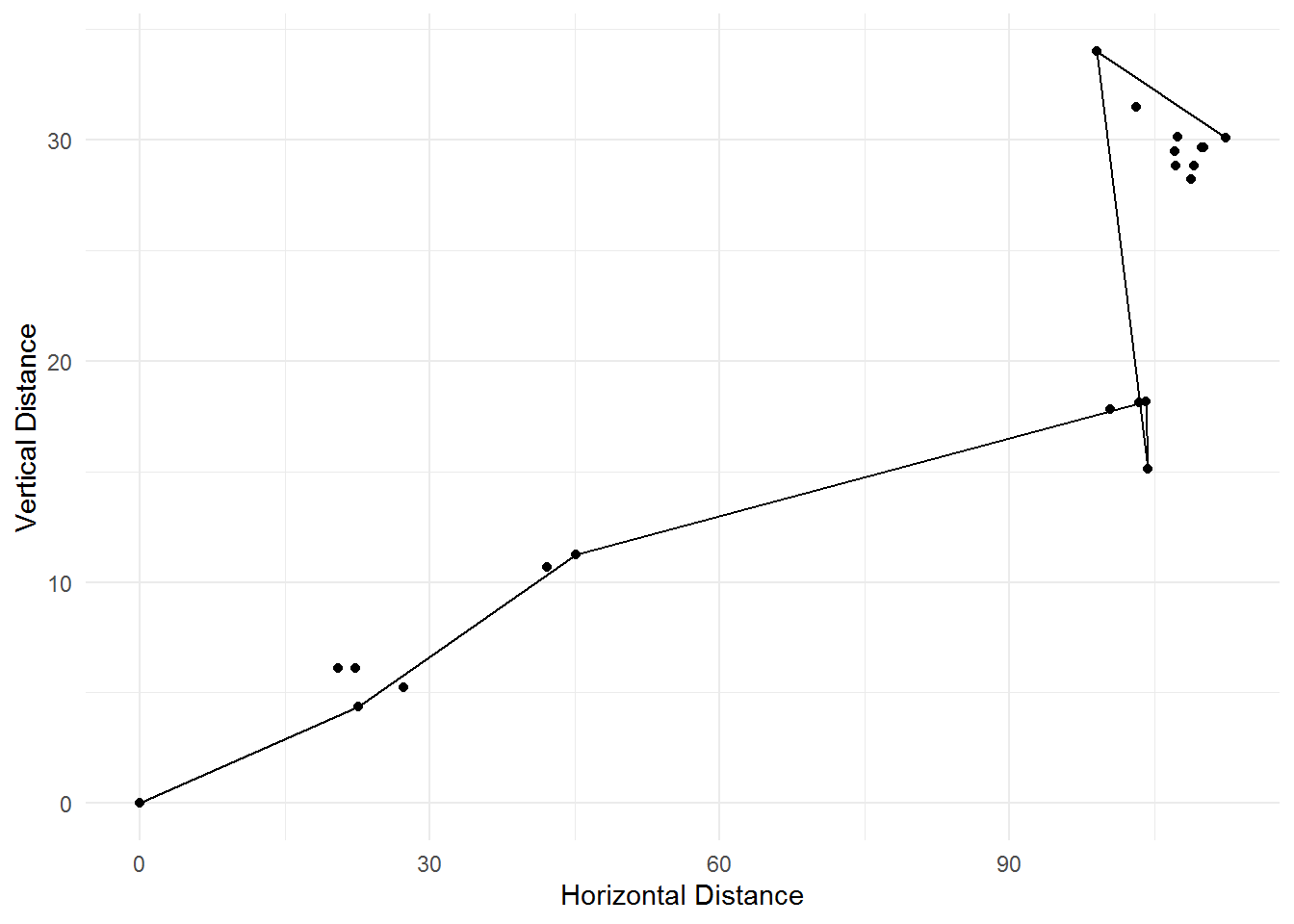Example of segmentation

# Algorithm

Methods of segmentation vary, but most follow along with the well-known Douglas-Peucker algorithm’s method of creating new segments based on the magnitude of discrepancy between the proposed segment and the point that it should represent. This simulation study implements the Top-Down Time Ratio algorithm outlined in Han (2011). A segment is generated between the first location in a trajectory and the last location in the trajectory. Each recorded location point between the two segment ends is given a proposed new point along the generated segment, with respect to the elapsed time. The distance is calculated between the recorded point and this pseudo-sampled point. The point lying furthest from its segment is selected to form a new segment end, whereupon the process begins again.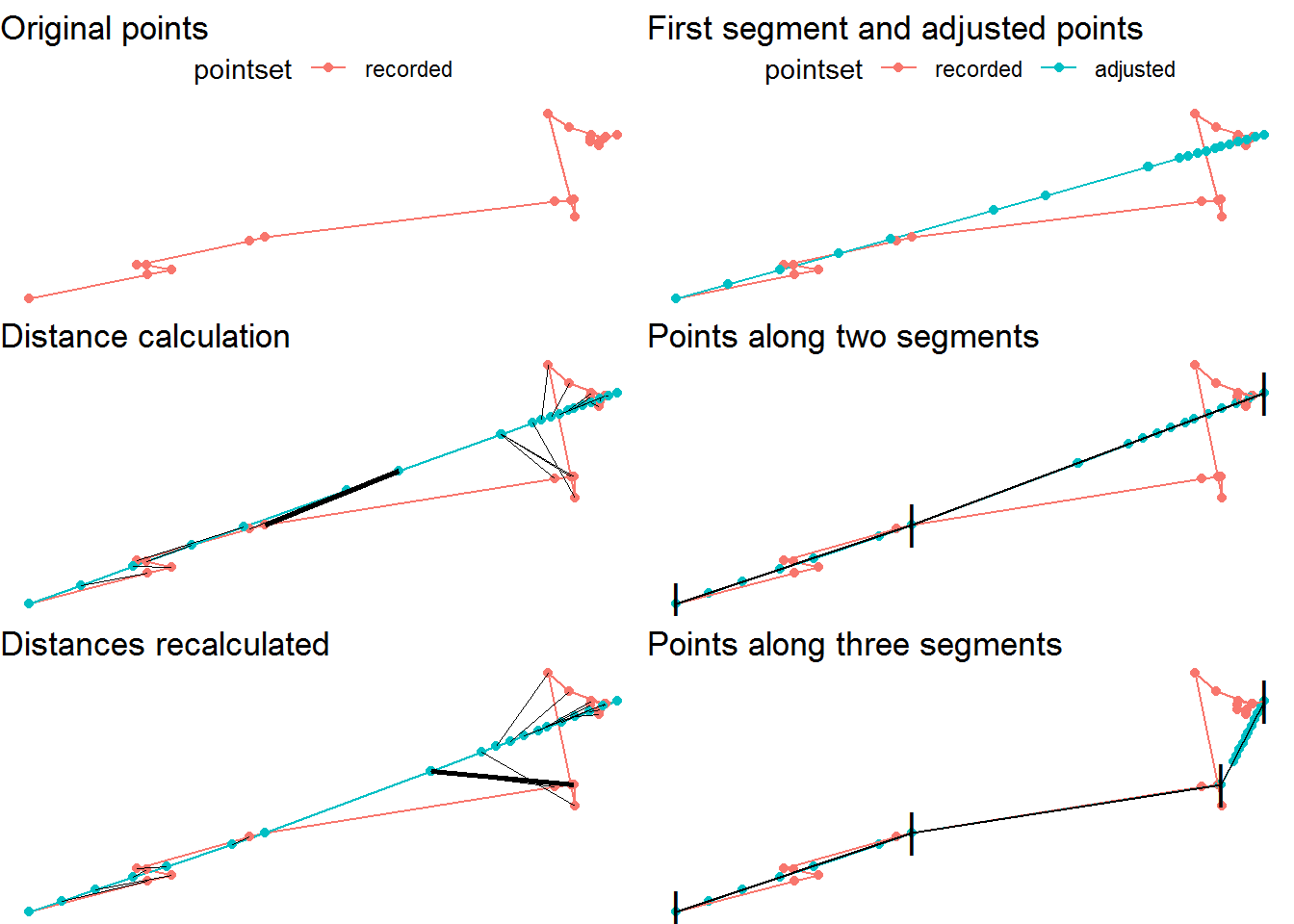# Simulation study

This simulation study was conducted on the set of data from the Tabi app that were complete for at least twenty-four consecutive hours. The distance covered as calculated by the summation of the distance between consecutive points was calculated for each individual set and used as a basis for comparison in order to determine the properties of the selected maximum error conditions. Error conditions ranged from a ten meter allowance to a 200 meter allowance by increments of ten.

Results from the simulation study in terms of total distance demonstrate that as expected, as the maximum allowed error increases, the total distance decreases. The overall change is modest in most cases and extreme in very few. Despite the wide range of error conditions, total distance as a whole remains largely stable even when accepting points that deviate by 200 meters. Total distance as a metric can give us a way to estimate the distance lost as we generate segments, but it is not in itself particularly informative.

Total distance in KM by Error Condition
Error Condition Min. 1st Qu. Median Mean 3rd Qu. Max.
200 0 22.4 62.9 137.5 167.7 3450.6
150 0 22.6 63.8 139.3 169.4 3451.5
100 0 23.6 65.2 141.2 171.1 3452.9
50 0 26.3 67.8 144.0 175.2 3454.3
30 0 27.8 69.9 146.5 178.4 3455.0
10 0 32.9 76.1 153.2 184.7 3456.7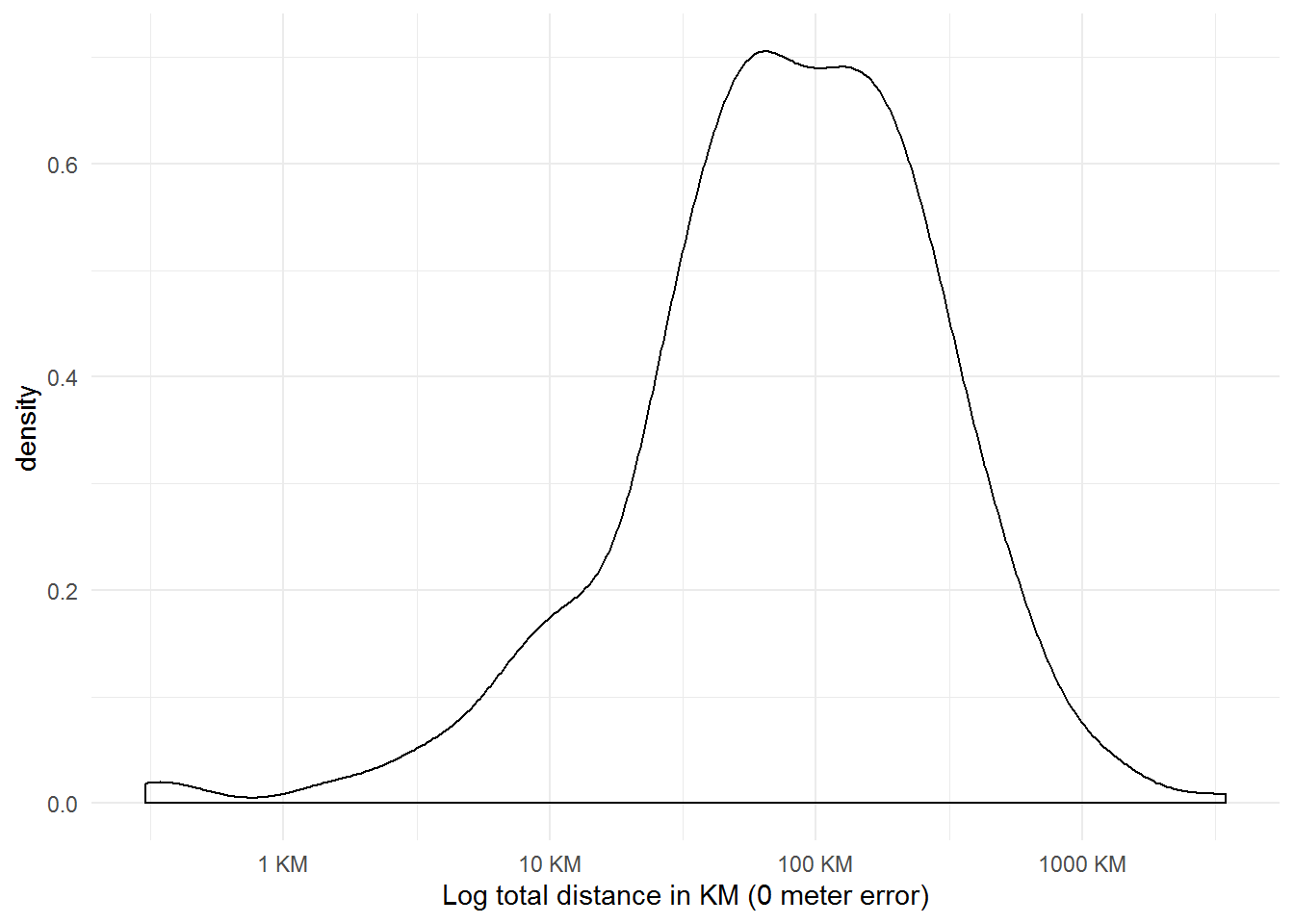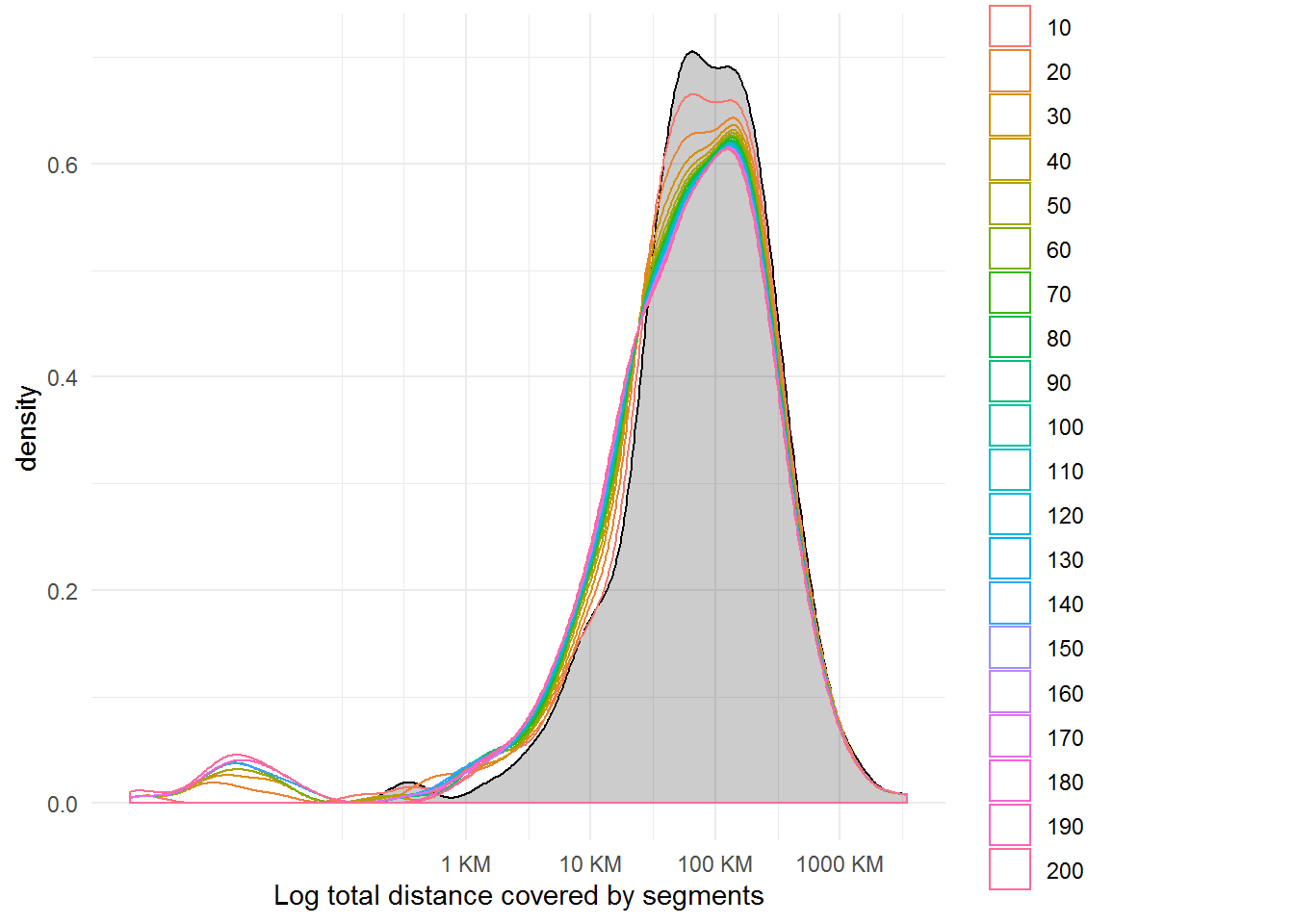Of interest is how the metrics calculated within a single data set might differ according to the selected criteria. We can investigate the deviance from the zero-meter error condition and its relationship with the total distance covered.

In the following figure, the difference in distance as a proportion of the total time is plotted against the error conditions. It becomes possible to discriminate between the levels of allowed error, although the results vary across data sets. In some sets, represented on the lower third of the figure, we see a gradually increasing line indicating a relatively smooth change from 10 meters max error towards 200 meters max error, and which end with a change in distance no greater than 10% of the total traveled distance. The other data sets respond relatively quickly to the change in error, but seem to reach a point of equilibrium before the 200 meter error condition. For these data sets, it may be very important to maintain a low max error.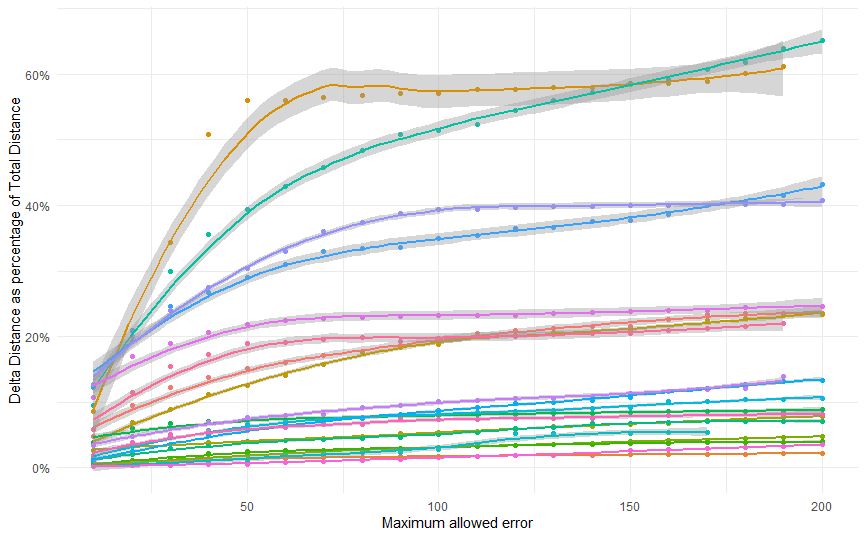proportion

The following figure shows the same relationship, but the maximum actual error is recorded in place of the maximum allowed error. Consider that we may reach a stopping point for a data set in the 100 meter error condition that is actually 95 meters at its greatest distance. If we consider the data in this way, we see largely the same results.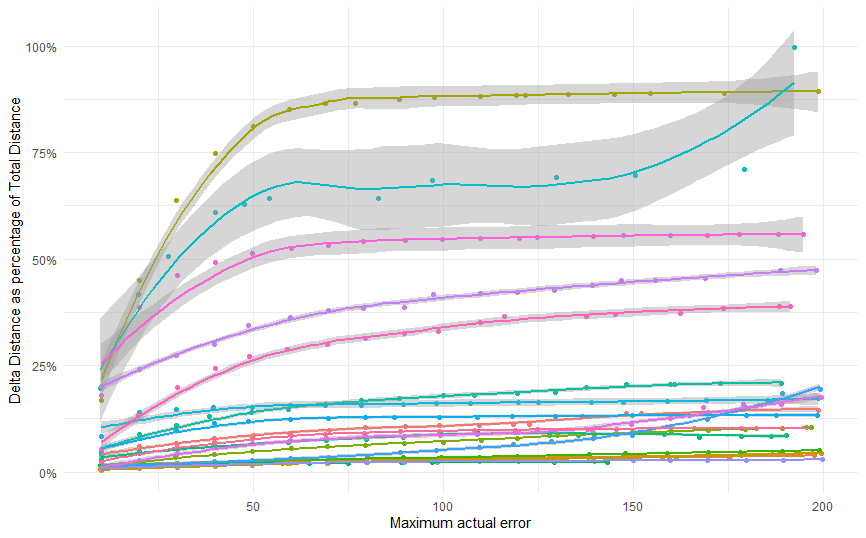It may also be of interest to look at the number of segments and mean error as it relates to the percentage of total distance as calculated in the zero-meter error condition. For some data sets, a relatively small number of segments is able to account for over 80% of the distance. But what about those data sets that never reach even 75% of the distance?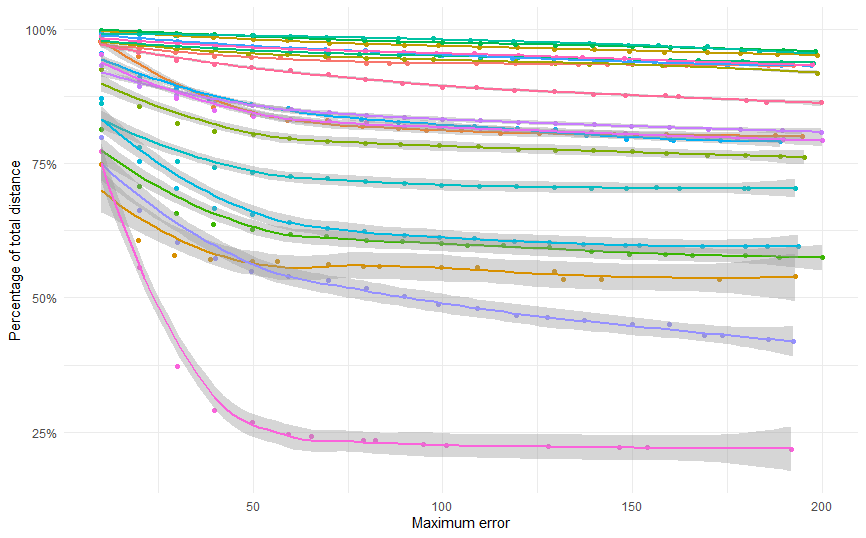maxerrperc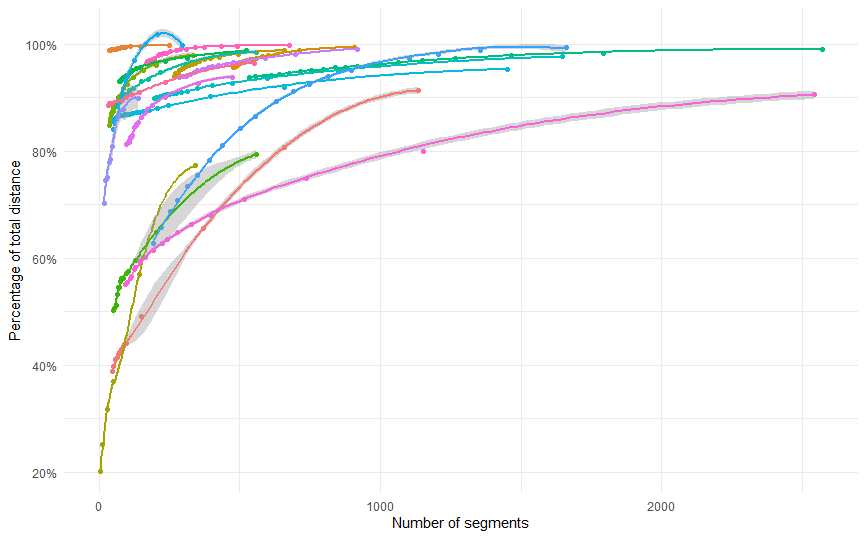Numberseg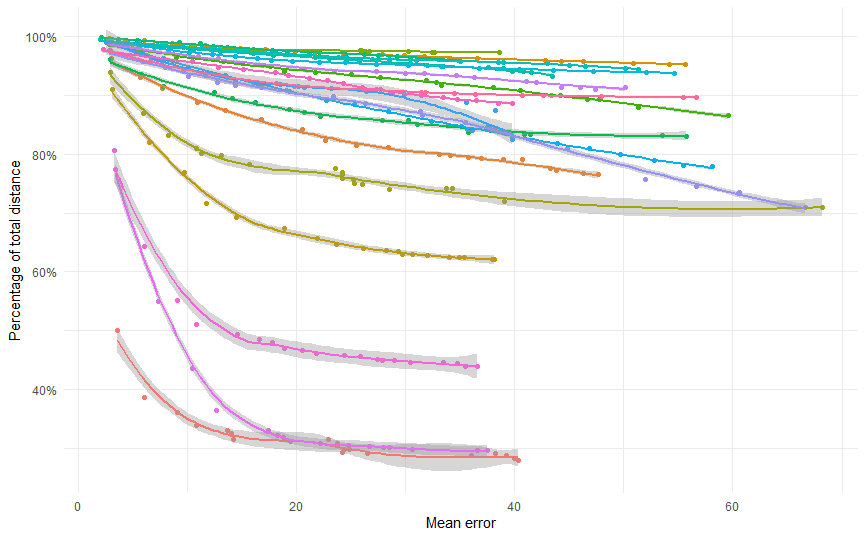meanerr

# Measurement error

Below are two examples of data sets in which even the 10m error condition misses a large percentage of the total distance. The segments drawn appear to be relatively faithful to the underlying path with only minor deviations (and in fact we see that the mean error is very low – around 2 meters). The recorded points are high-density over the time period which in the absence of error, would give us a very good estimation of total distance traveled. However, in this case, the high density of points coupled with a small amount of measurement error leads us to an overestimate of the distance traveled. The total distance of the continuous path is likely to fall somewhere between the 10 meter error and 0 meter error measurements.